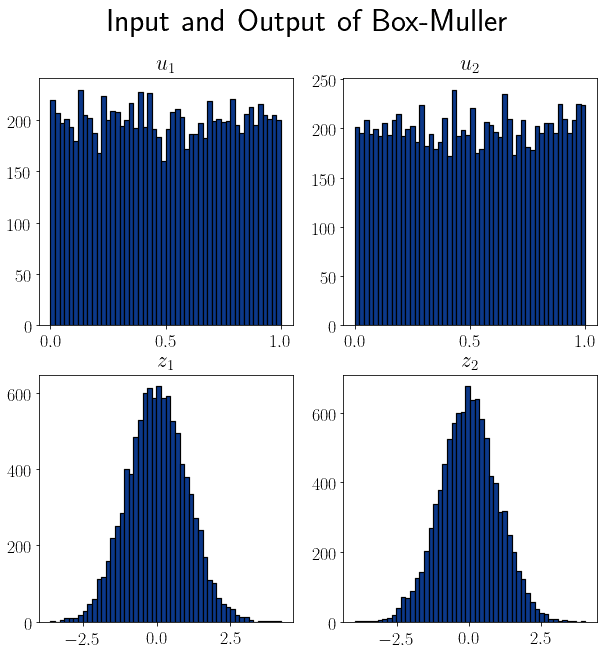## 1. Overview

In this tutorial, we’ll study how to convert a uniform distribution to a normal distribution.

We’ll first do a quick recap on the difference between the two distributions.

Then, we’ll study an algorithm, the Box-Muller transform, to generate normally-distributed pseudorandom numbers through samples from the uniform distribution.

At the end of this tutorial, we’ll know how to build a pseudorandom generator that outputs normally-distributed values.

## 2. Uniformity and Normality

The first point in this discussion is to understand how a uniform and normal distribution differ. This lets us concurrently understand what we need to transform one into the other and vice-versa.

### 2.1. The Uniform Distribution

The uniform distribution also takes the name of the rectangular distribution, because of the peculiar shape of its probability density function:Within any continuous interval, which may or not include the extremes, we can define a uniform distribution. This is the distribution for which all possible arbitrarily small intervals, with or without extremes, have the same probabilityof occurrence. We can verify that this is a proper probability desity function by calculating the area under the curve:### 2.2. The Normal Distribution

The normal distribution, instead, is a distribution characterized by this probability density function:In here,andindicate, respectively, the standard deviation and the mean of the distribution. This is its corresponding chart, forand:We can see that the values contained in a normal distribution aren’t equally likely, as the corresponding values of the uniform distribution were. This is due to two reasons.

The first reason is that the uniformly-distributed values with non-zero probability are all contained in a finite interval, while those of the normal distributions aren’t. We noted that the values with a non-zero probability in the normal distribution occupy the whole domain. This isn’t true for the uniform distribution, so we’ll need some kind of transformation to convert the finite intervalto the other.

The second reason is that all values in discrete uniform distributions have the same probability of being drawn. For discrete normal distributions, instead, any two valueshave corresponding probabilitiesdifferent from one another. Of course, with the exception of the case in which.

## 3. Box-Muller Transform

### 3.1. The Definition of the Algorithm

We can therefore identify an algorithm that maps the values drawn from a uniform distribution into those of a normal distribution. The algorithm that we describe here is the Box-Muller transform. This algorithm is the simplest one to implement in practice, and it performs well for the pseudorandom generation of normally-distributed numbers.

The algorithm is very simple. We first start with two random samples of equal length,and, drawn from the uniform distribution. Then, we generate from them two normally-distributed random variablesand. Their values are:

••### 3.2. Empirical Testing

We can empirically test whether the procedure grants us a normally distributed random variable or not. This is done, concretely, by plotting the histogram of a bivariate distributiondrawn from the uniform distribution. Then, we can compare it with the histogram of the corresponding transformed distributions:We made the charts above with two random samplesof size 10,000. We can see that the corresponding distributionshave the typical bell shape of a Gaussian distribution.

### 3.3. Deriving the Expression

We can understand how to derive the two expressions above if we imagine positioning the variablesin the Cartesian plane. There, each of them corresponds to one of the two coordinates of a point:Then, we can express the point in polar coordinates, identifying an angleand a distance offrom the origin. This is because a bivariate normal distributionhas a normthat corresponds to. Instead, the anglein the polar coordinates corresponds to.

Finally, this lets us map the two variablesin the original cartesian system to the normally-distributed variables, as:Lastly, we can easily notice how these expression are equivalent to the ones we gave above.

### 3.4. The Limitations of Box-Muller

This algorithm performs well if we use it to generate a relatively short sequence of normally-distributed values. For a sufficiently short sequence, in fact, we expect most of its numbers to be contained within three standard deviations of the distribution’s mean. If, however, the sequence is large, we expectof the values to be located outside of that interval.

In computers with finite accuracy for the representation of decimal digits, there’s a limit to how close to zero can we draw a number from the uniform distribution. This changes depending on whether we use double or floating-point precision, but still implies a non-zero resolution to our capacity to draw from a continuous uniform distribution.

As a consequence, we can’t represent all possible values from the normal distribution by using the Box-Muller algorithm, but only those in sufficient proximity of the mean. A good rule of thumb is to state that the tail of the distribution truncates atstandard deviations if we use 32-bits precision. If we use 64-bit precision instead, we can expect the generated values to be located withinstandard deviations.

### 3.5. Alternatives to Box-Muller

Alternatively, we can use other, more complex algorithms if we need to obviate the limitations of Box-Muller.

The first is the Ziggurat Algorithm, which uses the rejection sampling principle and generates normally-distributed values on the basis of a random seed and a lookup table.

The second is the Ratio-of-Uniforms, which demands the storage in memory of several double-precision values, but is otherwise computationally fast and simple.

The third is to calculate the inverse function to the cumulative distribution function for a normal distribution. This corresponds to calculating the quantile function of a normal distribution which, for standard normal distributions, coincides with the probit function.

## 4. Conclusion

In this tutorial, we studied how to generate a pseudorandom variable that’s distributed normally.

We did so by sampling from a bivariate uniform distribution and then applying the Box-Muller transform on them.